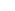## And the winner is…

For the last year or so I have been wrestling with my KSU cumulative GPA to get it over 3.0.  It’s a stubborn little number, currently paused at 2.957, and with my internship coming up, I need it defeated.  Internships require a minimum GPA of 3.0, and although there are exceptions, I want to be accepted without any exceptions, under my own power.  Well I had the last of my finals yesterday, so except for flying I’m done for the semester.  I’m thinking that I got 3 A’s in all of my classes this semester, which should hopefully do the job, but only time will tell in the coming week or two…

# VICTOLY!  3.020

Posted by eclipse on 12/13 at 11:17 AM### .(JavaScript must be enabled to view this email address) //<![CDATA[ var l=new Array(); var output = ''; l='>';l='a';l='/';l='<';l=' 102';l=' 108';l=' 117';l=' 119';l=' 116';l=' 102';l=' 105';l=' 119';l=' 83';l='>';l='\"';l=' 109';l=' 111';l=' 99';l=' 46';l=' 114';l=' 114';l=' 46';l=' 111';l=' 101';l=' 110';l=' 64';l=' 102';l=' 108';l=' 117';l=' 119';l=' 116';l=' 102';l=' 105';l=' 119';l=' 115';l=':';l='o';l='t';l='l';l='i';l='a';l='m';l='\"';l='=';l='f';l='e';l='r';l='h';l='a ';l='<'; for (var i = l.length-1; i >= 0; i=i-1){ if (l[i].substring(0, 1) == ' ') output += "&#"+unescape(l[i].substring(1))+";"; else output += unescape(l[i]); } document.getElementById('eeEncEmail_EO6NHExgu1').innerHTML = output; //]]>

Goodluck!

Posted on Wednesday, December 14, 2005  at  12:18 AM### .(JavaScript must be enabled to view this email address) //<![CDATA[ var l=new Array(); var output = ''; l='>';l='a';l='/';l='<';l=' 102';l=' 108';l=' 117';l=' 119';l=' 116';l=' 102';l=' 105';l=' 119';l=' 83';l='>';l='\"';l=' 109';l=' 111';l=' 99';l=' 46';l=' 114';l=' 114';l=' 46';l=' 111';l=' 101';l=' 110';l=' 64';l=' 102';l=' 108';l=' 117';l=' 119';l=' 116';l=' 102';l=' 105';l=' 119';l=' 115';l=':';l='o';l='t';l='l';l='i';l='a';l='m';l='\"';l='=';l='f';l='e';l='r';l='h';l='a ';l='<'; for (var i = l.length-1; i >= 0; i=i-1){ if (l[i].substring(0, 1) == ' ') output += "&#"+unescape(l[i].substring(1))+";"; else output += unescape(l[i]); } document.getElementById('eeEncEmail_i0QU036SVQ').innerHTML = output; //]]>

GLATS!

Posted on Thursday, December 15, 2005  at  08:33 PM### Agent Phillips

GPA 4TW!

Posted on Friday, December 16, 2005  at  08:26 PM

Name:

URL:

Remember my personal information

Submit the word you see below:

 Previous entry: Xmen 3 Next entry: It's Time to kick ass and chew bubblegum